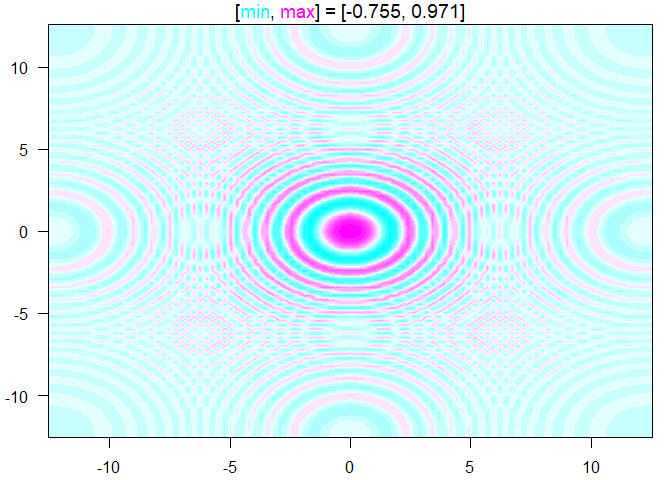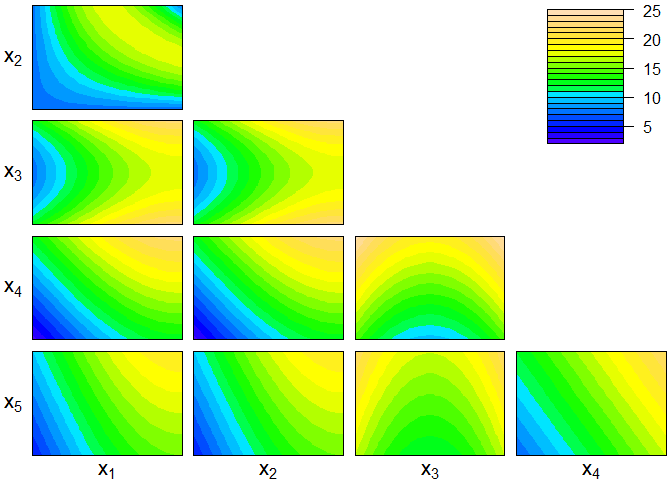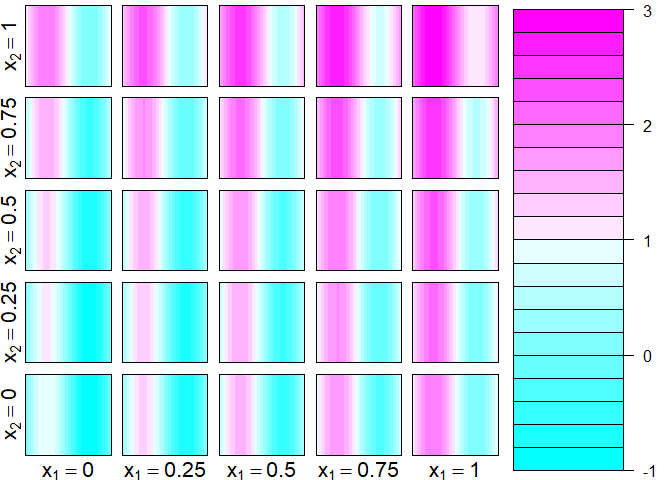# ContourFunctions

This is an R package that provides simple functions for creating contour plots.

## Overview

The main functions are:

• `cf_grid`: Makes a contour plot from grid data.

• `cf_func`: Makes a contour plot for a function.

• `cf_data`: Makes a contour plot for a data set by fitting a Gaussian process model.

• `cf`: Passes arguments to `cf_function` or `cf_data` depending on whether the first argument is a function or numeric.

All of these functions make the plot using base graphics by default. To make plots using ggplot2, add the argument `gg=TRUE`, or put g in front of the function name. E.g., `gcf_data(...)` is the same as `cf_data(..., gg=TRUE)`, and makes a similar plot to `cf_data` but using ggplot2.

There are two functions for making plots in higher dimensions:

• `cf_4dim`: Plots functions with four inputs by making a series of contour plots.

• `cf_highdim`: Plots for higher dimensional inputs by making a contour plot for each pair of input dimensions and holding the other inputs constant or averaging over them.

## Installation

``````# It can be installed like any other package
install.packages("ContourFunctions")

# Or the the development version from GitHub:
# install.packages("devtools")
devtools::install_github("CollinErickson/contour")``````

## Usage

Plot a grid of data:

``````library(ContourFunctions)
a <- b <- seq(-4*pi, 4*pi, len = 27)
r <- sqrt(outer(a^2, b^2, "+"))
cf_grid(a, b, cos(r^2)*exp(-r/(2*pi)))``````Plot a function with two input dimensions:

``````f1 <- function(r) cos(r^2 + r^2)*exp(-sqrt(r^2 + r^2)/(2*pi))
cf_func(f1, xlim = c(-4*pi, 4*pi), ylim = c(-4*pi, 4*pi))``````Using data with two inputs and an output, fit a Gaussian process model and show the contour surface with dots where the points are:

``````set.seed(0)
x <- runif(20)
y <- runif(20)
z <- exp(-(x-.5)^2-5*(y-.5)^2)
cf_data(x,y,z)``````For more than two input dimensions:

``````friedman <- function(x) {
10*sin(pi*x*x) + 20*(x-.5)^2 + 10*x + 5*x
}
cf_highdim(friedman, 5, color.palette=topo.colors)``````For (three or) four inputs dimensions:

``cf_4dim(function(x) {x + x^2 + sin(2*pi*x)})``